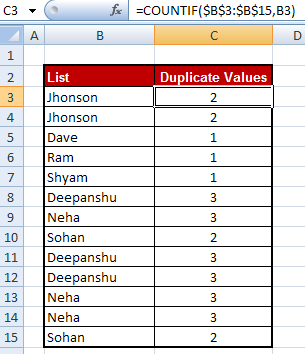# How to Count Duplicate Values in Excel

This tutorial explains how to count duplicate values in Excel, along with examples.

Counting duplicate values can help you to improve your data analysis. For example, if you are trying to summarize your data and there are duplicate values in the data, the summary statistics may be negatively impacted. Counting duplicate values can help you to avoid this problem.

Sample Data

Suppose you have some names in cells B3:B15 (excluding header) as shown in the image below.Count Duplicate Values

## How to Count Duplicate Values in Excel

The following formula calculates the count of duplicate values in the specified range. Here the range is B3:B15.

=SUMPRODUCT((1/COUNTIF(B3:B15,B3:B15)<1)*((1/COUNTIF(B3:B15,B3:B15))))

How it works :

• `COUNTIF(B3:B15,B3:B15)` returns the count of how many times the cell value appears in the range B3:B15. It does not return a single value but an array of the same size as the range (B3:B15).
• `1/COUNTIF(B3:B15,B3:B15)` takes the reciprocal of each value in the array from the previous step.
• `(1/COUNTIF(B3:B15,B3:B15)<1)` means that if a cell value appears more than once in the range, its corresponding element in this array will be TRUE, otherwise, it will be FALSE.
• `(1/COUNTIF(B3:B15,B3:B15))*((1/COUNTIF(B3:B15,B3:B15)<1))` multiplies the two arrays.
• Finally, `SUMPRODUCT((1/COUNTIF(B3:B15,B3:B15)<1)*((1/COUNTIF(B3:B15,B3:B15)<1)))` sums up all elements in the array from the previous step. In short it gives us the count of duplicates values in the range.
Formula : How to Check the Duplicate Values

Step I :

Paste =COUNTIF(\$B\$3:\$B\$15,B3) in cell C3 and paste it down till cell C15

Step II :

Apply filter on column C and uncheck 1. It filters duplicate values.
Related Posts